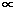# How Voltage affects Electric Power

• Electric Power is calculated by multiplying the current by the supply voltage:

• P (watts) = V (volts) x I (amps)

• The \$64 million dollar fact to remember from the previous slide is that the current is also influenced by voltage for most ordinary loads

• Therefore the customer's electric power consumption for many typical household and office loads is proportional to the square of the supply voltage.

• Mathematically this is written PV2

• Thus for many ordinary electrical loads, a six percent increase in voltage above 240 volts will by the application of Ohm's Law inevitably lead to a 12.4 per cent rise in the power consumption of the load.

How Voltage affects Electrical Energy Consumption
Michael Gunter http://www.suburbia.com.au/~mickgg